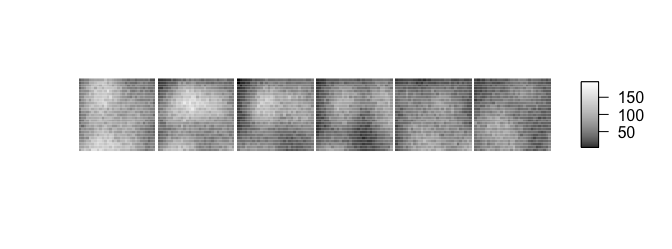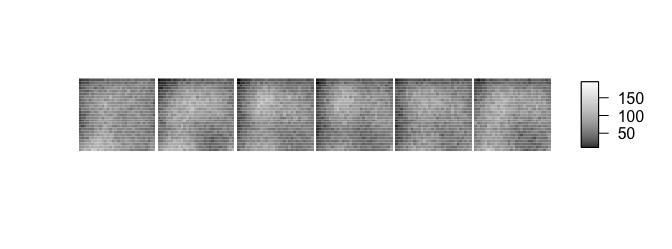# Detrending images with detrendr

#### 2018-02-17

Detrend image series.

library(detrendr)

## An image in need of detrending

The package contains a sample image series which can be found at
system.file("extdata", "bleached.tif", package = "detrendr"). It’s 500 frames of diffusing fluorescent particles which are bleaching over the course of the acquisition. We can see they’re bleaching by displaying every 99th frame.

library(magrittr)
path <- system.file("extdata", "bleached.tif", package = "detrendr")
img <- ijtiff::read_tif(path, msg = FALSE)
every100th <- purrr::map(seq(1, dim(img), by = 99), ~ img[, , 1, .]) %>%
purrr::reduce(~ cbind(.x, max(img), .y))
ijtiff::display(every100th)### Detrending

We see that the intensity is much lower for the last frame, this is because the image series has been bleached. We can correct for this.

system.time(corrected_exp <- img_detrend_exp(img, "auto", purpose = "FFS",
seed = 0, parallel = 2))["elapsed"]
#> elapsed
#>   2.422
every100th <- purrr::map(seq(1, dim(img), by = 99),
~ corrected_exp[, , 1, .]) %>%
purrr::reduce(~ cbind(.x, max(img), .y))
ijtiff::display(every100th)So we see that the corrected series does not have this drop-off in intensity.

Above we used exponential filtering detrending, but we could also use boxcar or polynomial.

system.time(corrected_boxcar <- img_detrend_boxcar(img, "auto", purpose = "FFS",
seed = 0, parallel = 2))["elapsed"]
#> elapsed
#>    0.94
system.time(corrected_polynom <- img_detrend_polynom(img, "auto",
purpose = "FFS",
seed = 0, parallel = 2))["elapsed"]
#> Warning in best_degree(img[, , i, ], seed = seed, parallel = parallel): The
#> polynomial degree found for your detrend was 17. Degrees above 3 are not
#> recommended as they usually indicate eccentric fits. It would be wise to
#> use another detrending method (exponential or boxcar).
#> elapsed
#>   4.849

Let’s check the mean brightness of each:

mean(brightness_pillars(corrected_exp))
#>  1.599681
mean(brightness_pillars(corrected_boxcar))
#>  1.449115
mean(brightness_pillars(corrected_polynom))
#>  1.597455

So we see that different methods give different results.